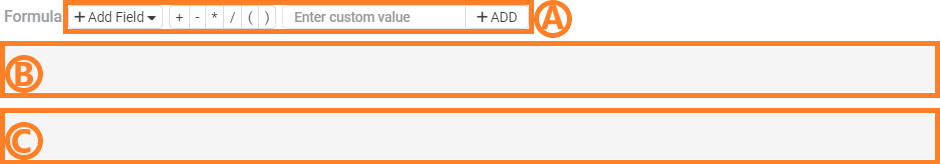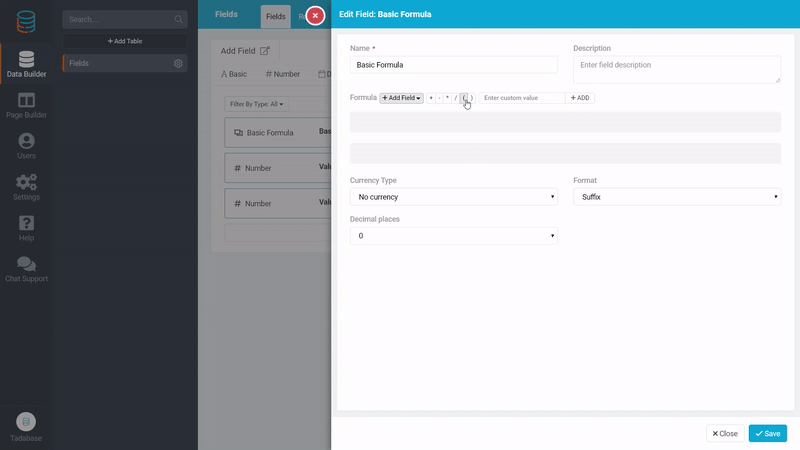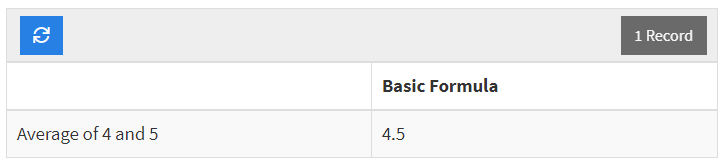# We have moved our support docs to a new location.

Basic Formula Fields are fields with automatically generated values based on a defined formula. These fields can reference other numeric values in their formula.1. The Name for your currency field will help define what this value represents.
2. Descriptions can be a good tool to keep track of further information to describe the field.
3. The Formula for the field must be defined for the value to be generated upon record creation/edit. Read ahead for further information on the Formula portion of this field.
4. The Currency Type can be selected based on the type of currency that you need to store.
5. The Format determines the location of the currency symbol in components.
6. Decimal Place values can be configured to show the specified number of digits after the decimal in your fields.

The Formula portion of the Basic Formula Field can be broken down into 3 key components as follows:1. Add the numeric value from a field in your record, an operation, or a custom numeric value.
2. Reorder or Delete components

To show a simple example of how this field can be used, let's walk through how you can create a Basic Formula Field that calculates the average of two numbers.We have a fairly simple table with 3 essential fields, Value 1, Value 2, and Basic Formula. Value 1 and Value 2 hold the two numbers for the calculation, and Basic Formula will store the calculated average. The field named Formula is used to create the "Average of.." statement seen in the image above. If you would like to learn more about how you can do this, please see our article on Text Formula FieldsNow we can configure the Formula. In the gif below, you can see how we can use the functionality of the Formula portion to configure the average formula for our example.Below is the resulting Formula.To test out our Formula, we'll create a simple form with Value 1 and Value 2.Lastly, we can confirm our results!